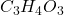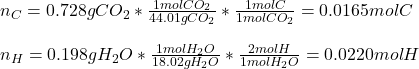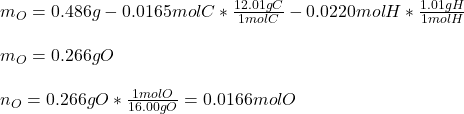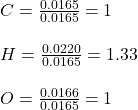## Vitamin C has the formula CxHyOz. You burn 0.486 g of the compound in a combustion analysis chamber and isolate 0.728 g of CO2 and 0.198 g o

Question

Vitamin C has the formula CxHyOz. You burn 0.486 g of the compound in a combustion analysis chamber and isolate 0.728 g of CO2 and 0.198 g of H2O. What is the empirical formula? Enter the elements in the order C, H, and O.

in progress 0
6 months 2021-07-30T00:24:05+00:00 2 Answers 4 views 0Explanation:

Hello there!

In this case, according to the given information derived from the combustion analysis, it turns out possible for us to realize that all the carbon comes from the CO2 and all the hydrogen from the H2O, it means we can calculate their moles in the vitamin C as shown below:Next, we calculate the grams and moles of oxygen from the grams of C and H in the sample:Then, we divide the moles of C, H, O by 0.0165 as the fewest moles in order to calculate the correct mole ratios:Finally, we turn them into whole number by multiplying by 3 so that the empirical formula is:Regards!

Relative atomic masses: C = 12.0, H = 1.0, O = 16.0

Mass fraction of C in CO₂ = 12.0/(12.0 + 16.0×2) = 12.0/44.0

Mass fraction of H in H₂O = 1.0×2/(1.0×2 + 18.0) = 2.0/18.0

In 0.486 g of the compound:

Mass of C = Mass of C in CO₂ = (0.728 g) × (12.0/44.0) = 0.199 g

Mass of H = Mass of H in H₂O = (0.198 g) × (2.0/18.0) = 0.022 g

Mass of O = (0.486 – 0.199 – 0.022) g = 0.265 g

x : y : z

= (0.199/12.0) : (0.022/1.0) : (0.265/16.0)

= 0.0166 : 0.022 : 0.0166

= (0.0166/0.0166) : (0.022/0.0166) : (0.0166/0.0166) … Divided by the smallest number

= 1 : 1.33 : 1

≈ 1 : (4/3) : 1

= 3 : 4 : 3

Hence, empirical formula = C₃H₄O₃